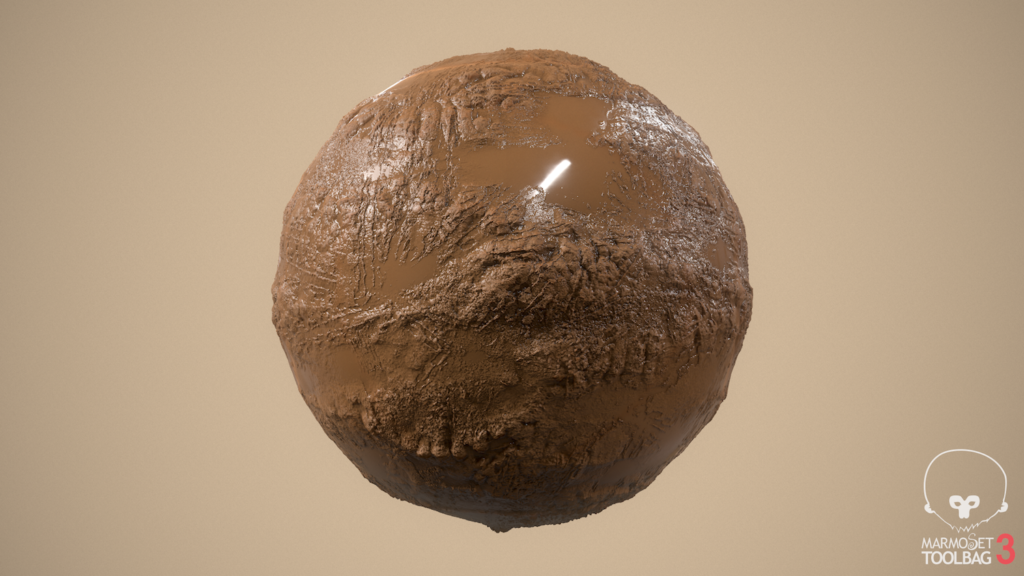# How can I make a “wet surface”/“shallow puddle” shader in Unity?

In my game I need to create dynamic water puddles but I cannot find a tutorial that shows how I can make such an effect (an example of which is shown below). How can I do it?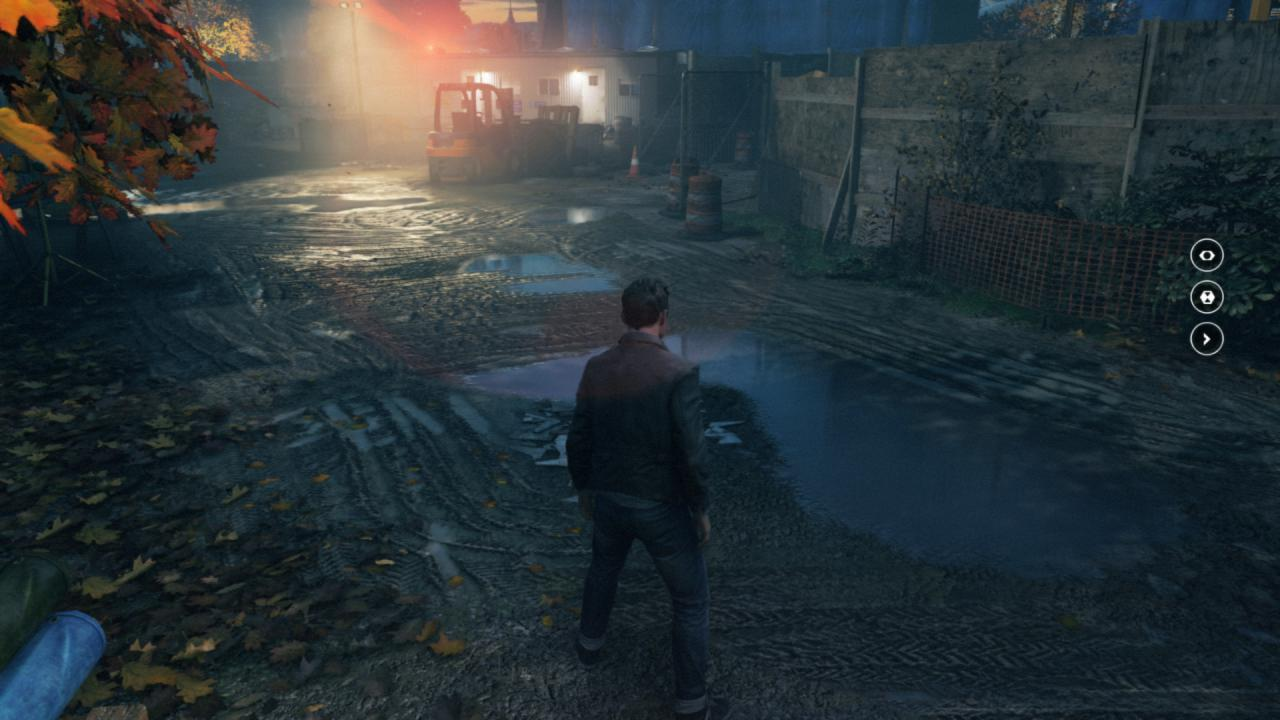• Annoying to see such a high voted question and higher voted answer not being closed. It is fine to choose your own answer as best, though a little silly to claim the bounty for yourself :) – Tim Holt Feb 2 '18 at 6:52
• @TimHolt On what basis would we close a question like this though? It seems perfectly on-topic. – user1430 Feb 7 '18 at 17:38
• I'm saying that the person who asked it should accept his own answer. Pardon my misuse of English. – Tim Holt Feb 8 '18 at 5:20

# Reflection

To create a wet shader, you first need to have a reflection.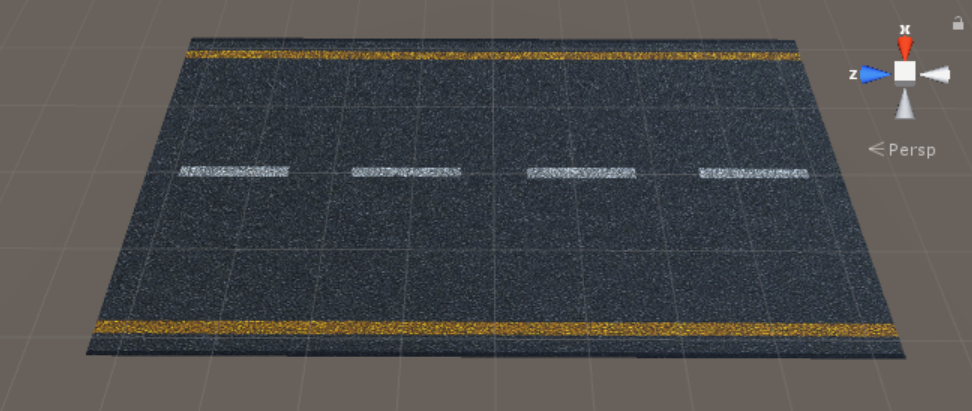You can use a Reflection Probe or a MirrorReflection3 but, I use a fake reflection (Cube Map) here because the shader can then be used on mobile.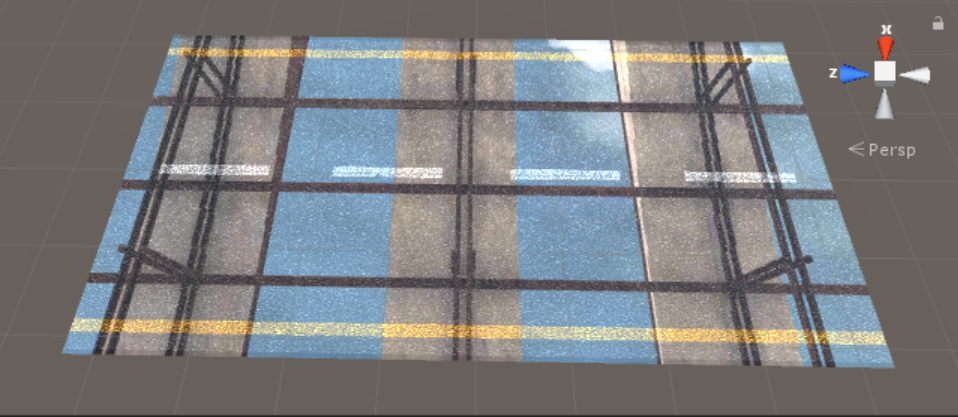Shader "Smkgames/TransparentCubeMap" {
Properties {
_Color("Color",Color) = (1,1,1,1)
_Cube ("Cubemap", CUBE) = "" {}
_Metallic("Metallic",Range(0,1)) = 1
_Smoothness("Smoothness",Range(0,1)) = 1
_Alpha("Alpha",Range(0,1)) = 1
}
Tags {"RenderType"="Transparent" "Queue"="Transparent"}
LOD 200
Pass {
}
ZWrite Off
Blend SrcAlpha OneMinusSrcAlpha

CGPROGRAM

struct Input {
float2 uv_MainTex;
float3 worldRefl;
};
sampler2D _MainTex;
samplerCUBE _Cube;
float4 _Color;
float _Metallic;
float _Smoothness;
float4 _EmissionColor;
float _Alpha;
void surf (Input IN, inout SurfaceOutputStandard o) {
fixed4 c = tex2D (_MainTex, IN.uv_MainTex) * _Color;

o.Albedo = c.rgb * 0.5 * _Color;
o.Emission = texCUBE (_Cube, IN.worldRefl).rgb*_Color;
o.Metallic = _Metallic;
o.Smoothness = _Smoothness;
o.Alpha = _Alpha;

}
ENDCG
}
Fallback "Diffuse"
}


# Distortion

To add distortion to your reflection, you can multiply the normal map and the worldRefl:

float3 distortion = tex2D(_Distortion, IN.uv_Distortion);
o.Emission = texCUBE(_Cube, IN.worldRefl*distortion).rgb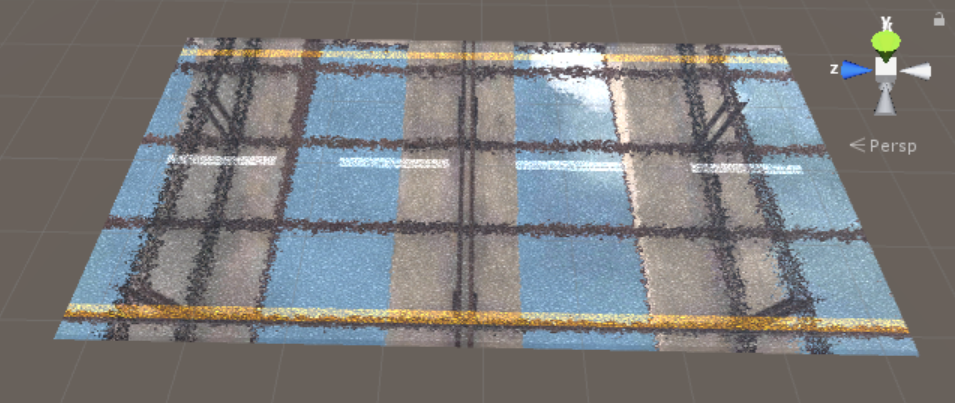# Procedural Shape

You can use noise to make a procedural shape: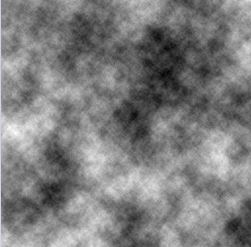Shader "Smkgames/FbmNoise"
{
Properties
{
_TileAndOffset("Tile and Offset",Vector) = (1,1,0,0)
}
{
Tags { "RenderType"="Opaque" }
LOD 100

Pass
{
CGPROGRAM
#pragma vertex vert
#pragma fragment frag
// make fog work
#pragma multi_compile_fog

#include "UnityCG.cginc"

struct appdata
{
float4 vertex : POSITION;
float2 uv : TEXCOORD0;
};

struct v2f
{
float2 uv : TEXCOORD0;
UNITY_FOG_COORDS(1)
float4 vertex : SV_POSITION;
};

float4 _TileAndOffset;
float _Step,_Min,_Ma;

v2f vert (appdata v)
{
v2f o;
o.vertex = UnityObjectToClipPos(v.vertex);
o.uv = v.uv*_TileAndOffset.xy+_TileAndOffset.zw;
UNITY_TRANSFER_FOG(o,o.vertex);
return o;
}

// Author @patriciogv - 2015
// http://patriciogonzalezvivo.com

float random (in float2 st) {
return frac(sin(dot(st.xy,
float2(12.9898,78.233)))*
43758.5453123);
}

// Based on Morgan McGuire @morgan3d
float noise (in float2 st) {
float2 i = floor(st);
float2 f = frac(st);

// Four corners in 2D of a tile
float a = random(i);
float b = random(i + float2(1.0, 0.0));
float c = random(i + float2(0.0, 1.0));
float d = random(i + float2(1.0, 1.0));

float2 u = f * f * (3.0 - 2.0 * f);

return lerp(a, b, u.x) +
(c - a)* u.y * (1.0 - u.x) +
(d - b) * u.x * u.y;
}

#define OCTAVES 6
float fbm (in float2 st) {
// Initial values
float value = 0.0;
float amplitude = .5;
float frequency = 0.;
//
// Loop of octaves
for (int i = 0; i < OCTAVES; i++) {
value += amplitude * noise(st);
st *= 2.;
amplitude *= .5;
}
return value;
}

fixed4 frag (v2f i) : SV_Target
{

float2 st =i.uv;

float3 color = float3(0,0,0);
color += fbm(st*3.0);
return float4(color,1.0);

}
ENDCG
}
}
}


The FBM above shouldn't be used directly in to your shader because it has many GPU calculations and decreases performance. Instead of using directly, you can render the result to a texture with RenderTexture.

Shadertoy uses multiple passes, one per "buffer". As the name indicates, this pass stores the results in a buffer, which is just a texture. Unity will let you render to textures too.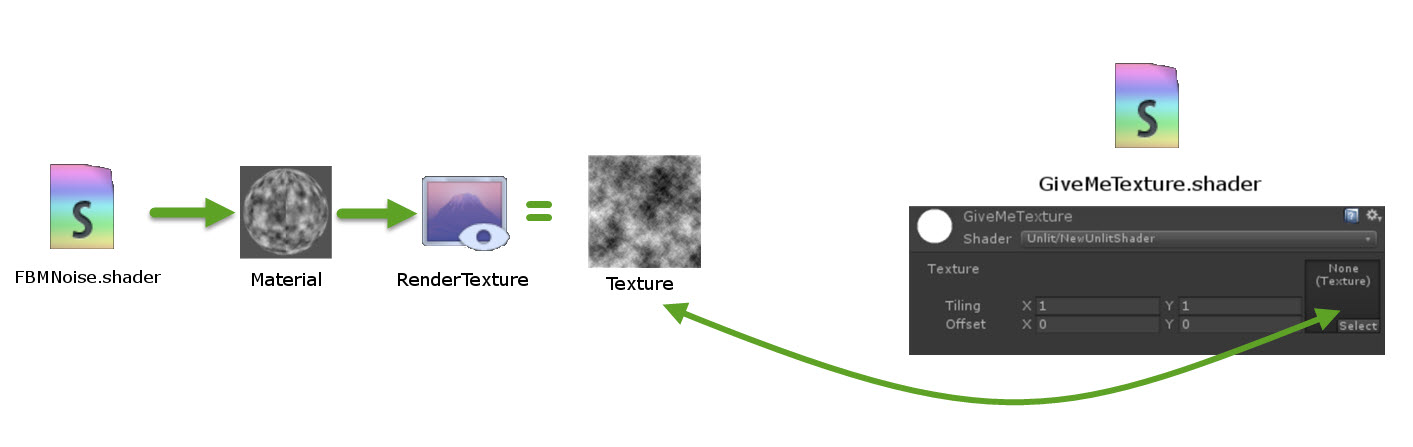You can make a thick and smooth mask with these functions:

Step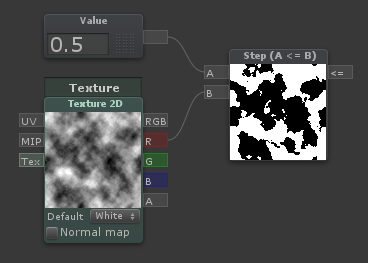Outputs 1 if [A] is less than or equal to [B], otherwise outputs 0.

Smoothstep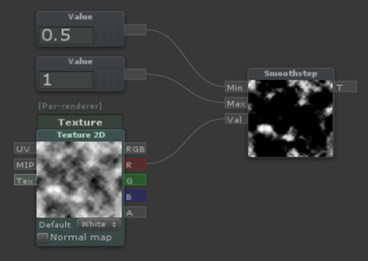Blends smoothly between two values, based on where a third value is in that range, outputting values between 0 and 1. Think of it as a clamped inverse lerp with a smoothed output value.

# Result

/* Warning: don't use this shader because this is for preview only.
It has many GPU calculations so if you want use this in your game you should
remove the FBM noise functions or render it to texture, or you can use an FBM texture
*/
//Created By Seyed Morteza Kamaly
Properties{
_MainTex("MainTex",2D) = "white"{}
_Distortion("Distortion",2D) = "bump"{}
_Cube("Cubemap", CUBE) = "" {}
_BumpMap("Bumpmap", 2D) = "bump" {}
_Metallic("Metallic",Range(0,1)) = 0
_Smoothness("Smoothness",Range(0,1)) = 1
_ReflectAlpha("ReflectAlpha",Range(0,1)) = 1
scaleX("UV.X scale",Float) = 10.0
scaleY("UV.Y scale",Float) = 10.0
_Smooth("Smooth",Float) = 0.4
_Intensity("Intensity",Float) = 1
}
Tags{ "RenderType" = "Transparent" "Queue" = "Transparent" }
LOD 200
Pass{
}
ZWrite Off
Blend SrcAlpha OneMinusSrcAlpha

CGPROGRAM

struct Input {
float2 uv_MainTex;
float2 uv_Distortion;
float3 worldRefl;
float2 uv_BumpMap;
INTERNAL_DATA
};
sampler2D _MainTex, _Distortion;
samplerCUBE _Cube;
float _Metallic,_Smoothness;
float4 _EmissionColor;
sampler2D _NormalMap;
uniform fixed scaleX, scaleY, _Smooth, _Intensity,_Alpha,_ReflectAlpha;

static const float2x2 m = float2x2(-0.5, 0.8, 1.7, 0.2);

float hash(float2 n)
{
return frac(sin(dot(n, float2(95.43583, 93.323197))) * 65536.32);
}

float noise(float2 p)
{
float2 i = floor(p);
float2 u = frac(p);
u = u*u*(3.0 - 2.0*u);
float2 d = float2 (1.0, 0.0);
float r = lerp(lerp(hash(i), hash(i + d.xy), u.x), lerp(hash(i + d.yx), hash(i + d.xx), u.x), u.y);
return r*r;
}

float fbm(float2 p)
{
float f = 0.0;
f += 0.500000*(0.5 + 0.5*noise(p));
return f;
}

float fbm2(float2 p)
{
float f = 0.0;
f += 0.500000*(0.6 + 0.45*noise(p)); p = p*2.02; p = mul(p, m);
f += 0.250000*(0.6 + 0.36*noise(p));
return f;
}

void surf(Input IN, inout SurfaceOutputStandard o) {
fixed4 c = tex2D(_MainTex, IN.uv_MainTex);

o.Metallic = _Metallic;
o.Smoothness = _Smoothness;
o.Alpha = 1;

float t = fbm2(float2(IN.uv_MainTex.x*scaleX, IN.uv_MainTex.y*scaleY));

float3 distortion = tex2D(_Distortion, IN.uv_Distortion);

o.Albedo = float4(1.0, 1.0, 1.0, 1.0)*tex2Dlod(_MainTex, float4(IN.uv_MainTex, 0.0, 0.0));

}
ENDCG
}
Fallback "Diffuse"
}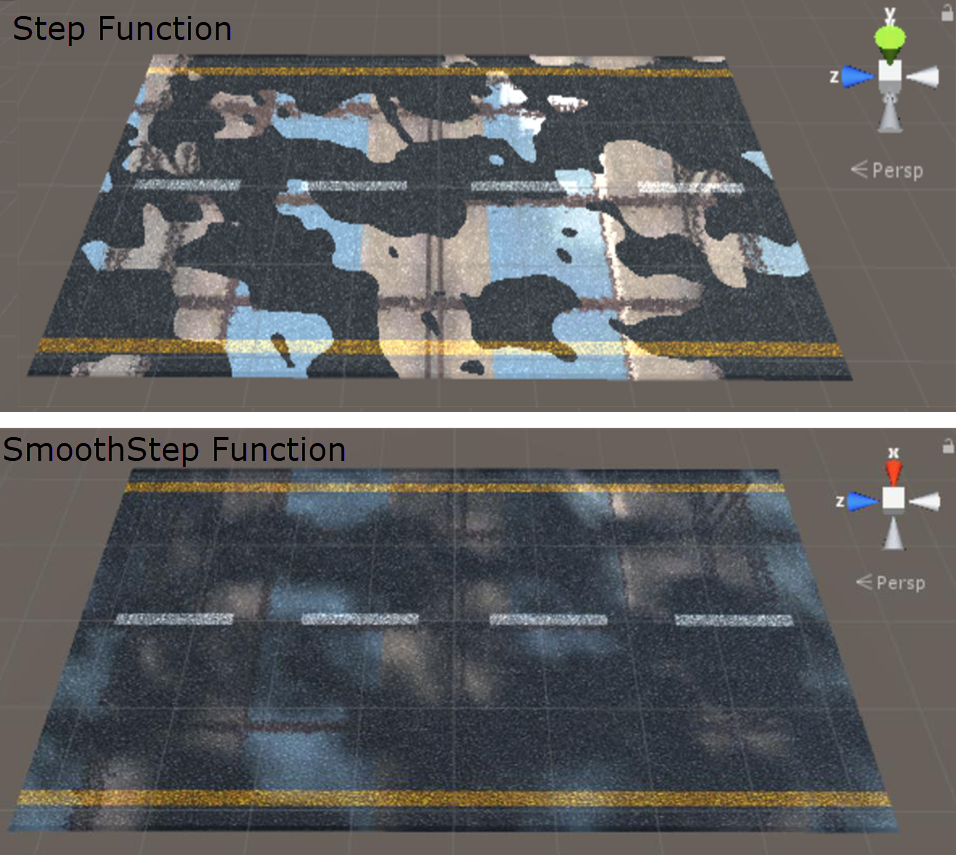# Using Maps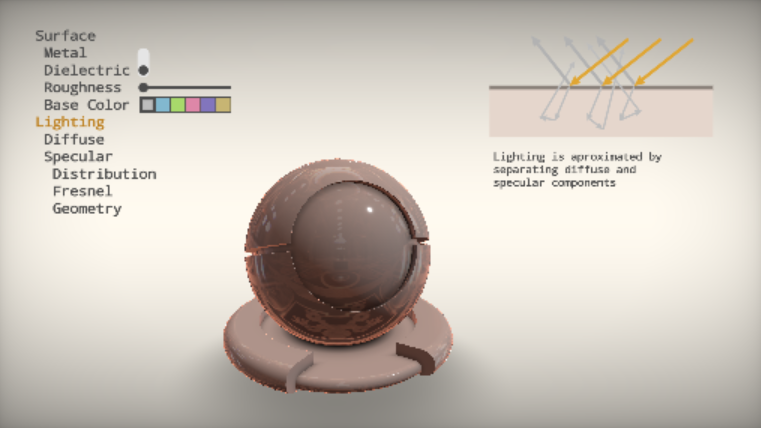Physically Based Shading

Here's a useful definitions:

Roughness Describes the microsurface of the object. White 1.0 is rough and black 0.0 is smooth. The microsurface if rough can cause the light rays to scatter and make the highlight appear dimmer and more broad. The same amount of light energy is reflected going out as coming into the surface. This map has the most artistic freedom. There is no wrong answers here. This map gives the asset the most character as it truly describes the surface e.g. scratches, fingerprints, smudges, grime etc.

Glossiness This map is the inverse of the roughness map. White 1.0 is smooth and 0.0 black is rough. Describes the microsurface of the object. The microsurface if rough can cause the light rays to scatter and make the highlight appear dimmer and more broad. The same amount of light energy is reflected going out as coming into the surface. This map has the most artistic freedom. There is no wrong answers here. This map gives the asset the most character as it truly describes the surface e.g. scratches, fingerprints, smudges, grime etc.

Specular This map contains the reflectance information for both metal and dielectrics (non metal) surfaces. This is a key difference in the metal/rough and spec/gloss workflows. The same rules apply. You need to use measured values for metals and most all dielectrics will fall with the 0.04 - 4% range. If there is dirt on the metal, the reflectance value needs to be lowered as well. However, you can add different values in the specular map for dielectric materials since you have control to author the map.

https://forum.allegorithmic.com/index.php?topic=3243.0

# Roughness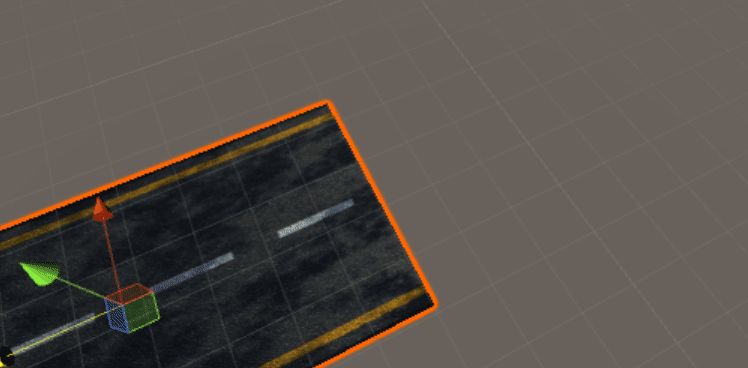I don't know why, but the Unity standard shader doesn't have a smoothness map, so I wrote a basic shader and added this map.

Shader "Smkgames/SimpleSurface" {
Properties {
_Color ("Color", Color) = (1,1,1,1)
_MainTex ("Albedo (RGB)", 2D) = "white" {}
_GlossMap("GlossMap",2D) = "white"{}
_Glossiness ("Smoothness", Float) = 1.5
_Metallic ("Metallic", Float) = 0.5
_MetallicMap("MetallicMap",2D) = "white"{}
}
Tags { "RenderType"="Opaque" }
LOD 200

CGPROGRAM

#pragma target 3.0

sampler2D _MainTex;

struct Input {
float2 uv_MainTex;
};

half _Glossiness,_Metallic;
fixed4 _Color;
sampler2D _GlossMap,_MetallicMap;

UNITY_INSTANCING_CBUFFER_START(Props)
UNITY_INSTANCING_CBUFFER_END

void surf (Input IN, inout SurfaceOutputStandard o) {
fixed4 c = tex2D (_MainTex, IN.uv_MainTex) * _Color;
o.Albedo = c.rgb;
o.Metallic = _Metallic*tex2D(_MetallicMap,IN.uv_MainTex);
o.Smoothness = _Glossiness*tex2D(_GlossMap,IN.uv_MainTex);
o.Alpha = c.a;
}
ENDCG
}
FallBack "Diffuse"
}


I think Unity doesn't have roughness, it only has metallic, but the alpha channel is for roughness and the red channel is for metallic. You can change the intensity with smoothness.